﻿ Python读写C语言数据结构 - ctypes 和 struct 库 - 蘭雅sRGB 个人笔记

C, C++, Python Programming, Source Code, Video

# Python读写C语言数据结构 - ctypes 和 struct 库## Python 的外部函数库 ctypes

• ctypes 是 Python 的外部函数库。它提供了与 C 兼容的数据类型，并允许调用 DLL 或共享库中的函数。可使用该模块以纯 Python 形式对这些库进行封装。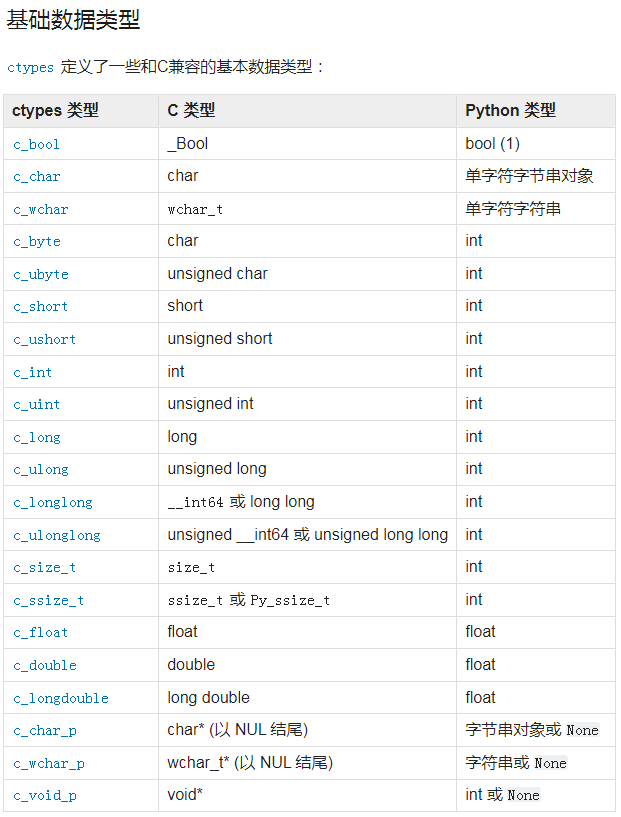### Python 的外部函数库 `ctypes` 之 结构体和联合

type 字段必须是一个 ctypes 类型，比如 c_int，或者其他 ctypes 类型: 结构体、联合、数组、指针。

``````from ctypes import *
class POINT(Structure):
_fields_ = [("x", c_int),
("y", c_int)]

point = POINT(10, 20)
print(point.x, point.y)

point = POINT(y=5)
print(point.x, point.y)

POINT(1, 2, 3)``````

## python读写C语言数据结构.py 实验源码

### C 语言定义的结构体 `struct gps` 在Python中对应写成 `class Location(Structure)`

``````struct gps {
double x; double y; };

class Location(Structure):
_fields_ = [('lon', c_double), ('lat', c_double)]``````

### pybin.py `Python` 使用 C数据结构，把一个gps坐标写到文件中。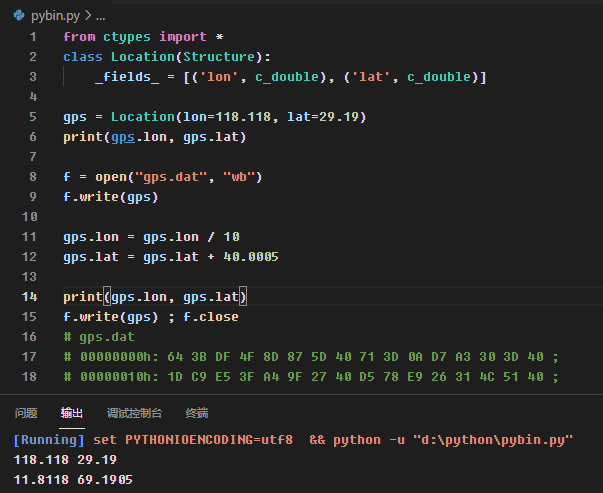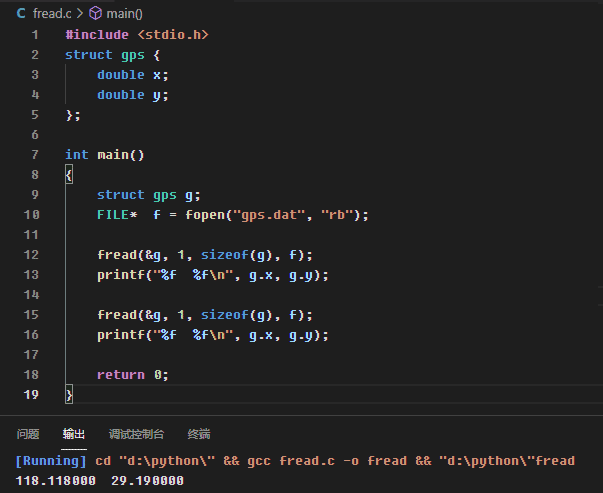### pybin_read.py `Python` 读取C数据结构文件后，需要使用 `struct` 库进行二进制数据转换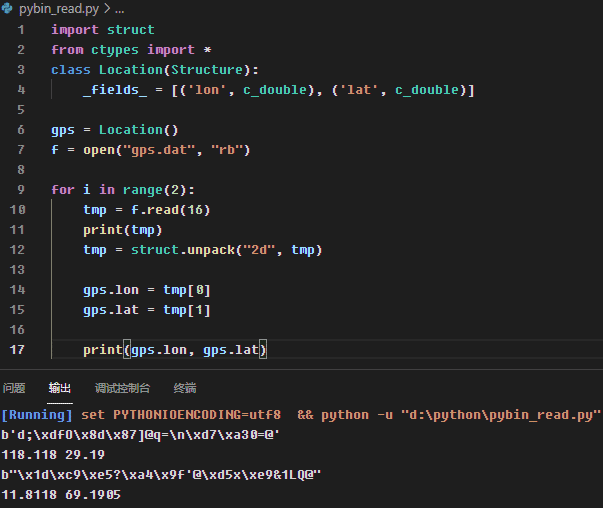## 将字节串解读为打包的二进制数据 struct

• 此模块可以执行 Python 值和以 Python bytes 对象表示的 C 结构之间的转换。
这可以被用来处理存储在文件中或是从网络连接等其他来源获取的二进制数据。

``````aa = struct.pack("4B", 1, 2, 3, 4)
print(aa)
bb = struct.unpack("f", aa)
print(bb)``````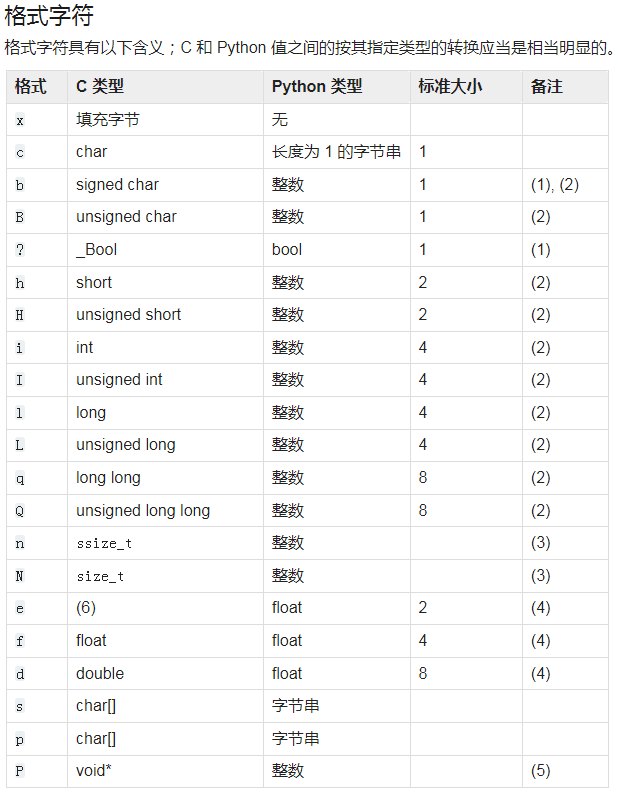## Python读写C语言数据结构 总结精简

``````import struct
from ctypes import *
class Location(Structure):
_fields_ = [('lon', c_double), ('lat', c_double)]

f = open("gps.dat", "rb")